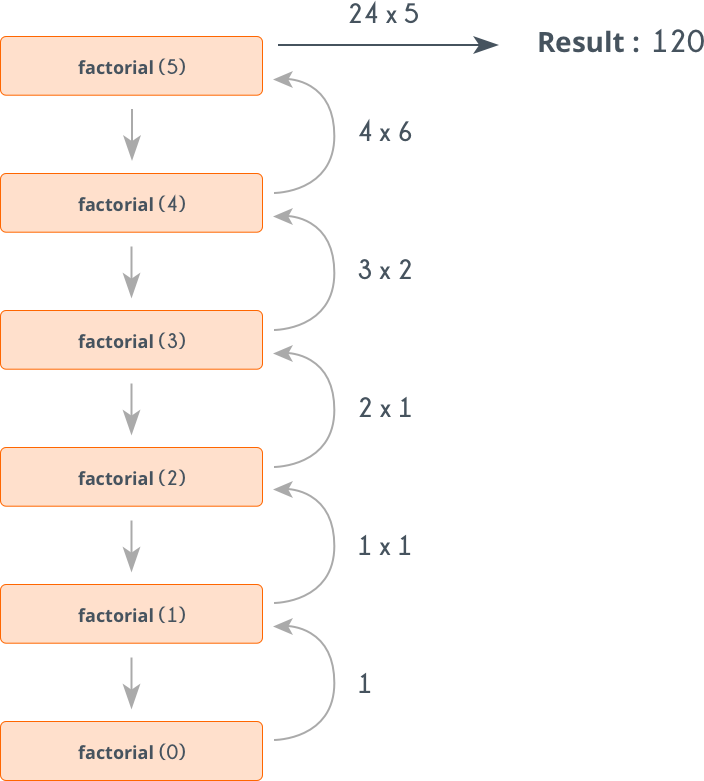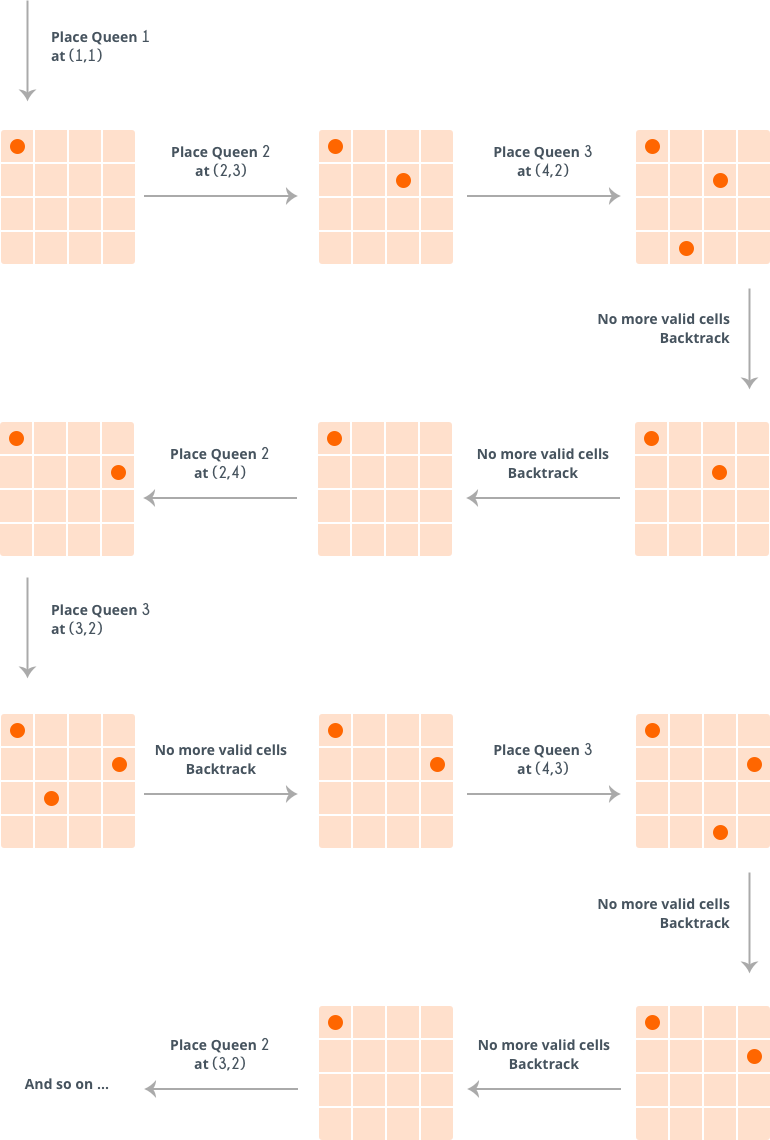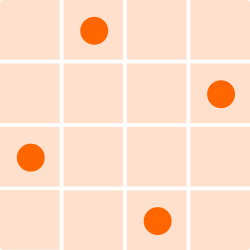Basic Programming
Topics:
Recursion and Backtracking
• Input/Output
• Complexity Analysis
• Implementation
• Operators
• Bit Manipulation
• Recursion
• Recursion and Backtracking

# Recursion and Backtracking

When a function calls itself, its called Recursion. It will be easier for those who have seen the movie Inception. Leonardo had a dream, in that dream he had another dream, in that dream he had yet another dream, and that goes on. So it's like there is a function called $dream()$, and we are just calling it in itself.

function dream()
print "Dreaming"
dream()


Recursion is useful in solving problems which can be broken down into smaller problems of the same kind. But when it comes to solving problems using Recursion there are several things to be taken care of. Let's take a simple example and try to understand those. Following is the pseudo code of finding factorial of a given number $X$ using recursion.

function factorial(x)
if x is 0                    // base case
return 1
return x*factorial(x-1)       // break into smaller problem(s)


The following image shows how it works for $factorial(5)$.Base Case: Any recursive method must have a terminating condition. Terminating condition is one for which the answer is already known and we just need to return that. For example for the factorial problem, we know that $factorial(0) = 1$, so when $x$ is 0 we simply return 1, otherwise we break into smaller problem i.e. find factorial of $x-1$. If we don't include a Base Case, the function will keep calling itself, and ultimately will result in stack overflow. For example, the $dream()$ function given above has no base case. If you write a code for it in any language, it will give a runtime error.

Number of Recursive calls: There is an upper limit to the number of recursive calls that can be made. To prevent this make sure that your base case is reached before stack size limit exceeds.

So, if we want to solve a problem using recursion, then we need to make sure that:

• The problem can broken down into smaller problems of same type.
• Problem has some base case(s).
• Base case is reached before the stack size limit exceeds.

## Backtracking:

So, while solving a problem using recursion, we break the given problem into smaller ones. Let's say we have a problem $A$ and we divided it into three smaller problems $B$, $C$ and $D$. Now it may be the case that the solution to $A$ does not depend on all the three subproblems, in fact we don't even know on which one it depends.
Let's take a situation. Suppose you are standing in front of three tunnels, one of which is having a bag of gold at its end, but you don't know which one. So you'll try all three. First go in tunnel $1$, if that is not the one, then come out of it, and go into tunnel $2$, and again if that is not the one, come out of it and go into tunnel $3$. So basically in backtracking we attempt solving a subproblem, and if we don't reach the desired solution, then undo whatever we did for solving that subproblem, and try solving another subproblem.

Let's take a standard problem.
N-Queens Problem: Given a chess board having $N \times N$ cells, we need to place $N$ queens in such a way that no queen is attacked by any other queen. A queen can attack horizontally, vertically and diagonally.

So initially we are having $N \times N$ unattacked cells where we need to place $N$ queens. Let's place the first queen at a cell $(i,j)$, so now the number of unattacked cells is reduced, and number of queens to be placed is $N-1$. Place the next queen at some unattacked cell. This again reduces the number of unattacked cells and number of queens to be placed becomes $N-2$. Continue doing this, as long as following conditions hold.

• The number of unattacked cells is not $0$.
• The number of queens to be placed is not $0$.

If the number of queens to be placed becomes $0$, then it's over, we found a solution. But if the number of unattacked cells become $0$, then we need to backtrack, i.e. remove the last placed queen from its current cell, and place it at some other cell. We do this recursively.
Complete algorithm is given below:

is_attacked( x, y, board[][], N)
//checking for row and column
if any cell in xth row is 1
return true
if any cell in yth column is 1
return true

//checking for diagonals
if any cell (p, q) having p+q = x+y is 1
return true
if any cell (p, q) having p-q = x-y is 1
return true
return false

N-Queens( board[][], N )
if N is 0                     //All queens have been placed
return true
for i = 1 to N {
for j = 1 to N {
if is_attacked(i, j, board, N) is true
skip it and move to next cell
board[i][j] = 1            //Place current queen at cell (i,j)
if N-Queens( board, N-1) is true    // Solve subproblem
return true                   // if solution is found return true
board[i][j] = 0            /* if solution is not found undo whatever changes
were made i.e., remove  current queen from (i,j)*/
}
}
return false

Here's how it works for $N=4$.So, at the end it reaches the following solution:So, clearly, the above algorithm, tries solving a subproblem, if that does not result in the solution, it undo whatever changes were made and solve the next subproblem. If the solution does not exists $(N = 2)$, then it returns $false$.

Contributed by: Vaibhav Jaimini
Уведомления

?